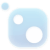# Top 20 NuGet blas Packages

The package includes dynamic win-x64 libraries and header files Intel® oneAPI Math Kernel Library (Intel® oneMKL) is a computing math library of highly optimized, extensively threaded routines for applications that require maximum performance. This package provides C and Data Parallel C++ (DPC++) p...
The package includes static win-x86 libraries Intel® oneAPI Math Kernel Library (Intel® oneMKL) is a computing math library of highly optimized, extensively threaded routines for applications that require maximum performance. This package provides C and Data Parallel C++ (DPC++) programming languag...
The package includes static win-x64 libraries Intel® oneAPI Math Kernel Library (Intel® oneMKL) is a computing math library of highly optimized, extensively threaded routines for applications that require maximum performance. This package provides C and Data Parallel C++ (DPC++) programming languag...
The package includes runtime win-x64 libraries Intel® oneAPI Math Kernel Library (Intel® oneMKL) is a computing math library of highly optimized, extensively threaded routines for applications that require maximum performance. This package provides C and Data Parallel C++ (DPC++) programming langua...
Intel® Math Kernel Library (Intel® MKL) is a computing math library of highly optimized, extensively threaded routines for applications that require maximum performance. This package provides C programming language interfaces. Intel MKL C language interfaces can be called from applications written i...
The package includes dynamic win-x86 libraries and header files Intel® oneAPI Math Kernel Library (Intel® oneMKL) is a computing math library of highly optimized, extensively threaded routines for applications that require maximum performance. This package provides C and Data Parallel C++ (DPC++) p...
The package includes dynamic win-x64 cluster libraries and header files Intel® oneAPI Math Kernel Library (Intel® oneMKL) is a computing math library of highly optimized, extensively threaded routines for applications that require maximum performance. This package provides C and Data Parallel C++ (...
The package includes static win-x64 cluster libraries Intel® oneAPI Math Kernel Library (Intel® oneMKL) is a computing math library of highly optimized, extensively threaded routines for applications that require maximum performance. This package provides C and Data Parallel C++ (DPC++) programming...
The package includes runtime win-x86 libraries Intel® oneAPI Math Kernel Library (Intel® oneMKL) is a computing math library of highly optimized, extensively threaded routines for applications that require maximum performance. This package provides C and Data Parallel C++ (DPC++) programming langua...
The package includes runtime win-x64 cluster libraries Intel® oneAPI Math Kernel Library (Intel® oneMKL) is a computing math library of highly optimized, extensively threaded routines for applications that require maximum performance. This package provides C and Data Parallel C++ (DPC++) programmin...
Package includes osx-x64 static libraries and interfaces Intel® oneAPI Math Kernel Library (Intel® oneMKL) is a computing math library of highly optimized, extensively threaded routines for applications that require maximum performance. This package provides C and Data Parallel C++ (DPC++) programm...
Package includes linux-x86 static libraries and interfaces Intel® oneAPI Math Kernel Library (Intel® oneMKL) is a computing math library of highly optimized, extensively threaded routines for applications that require maximum performance. This package provides C and Data Parallel C++ (DPC++) progra...
Package includes osx-x64 static libraries and interfaces for distributed memory function domains Intel® oneAPI Math Kernel Library (Intel® oneMKL) is a computing math library of highly optimized, extensively threaded routines for applications that require maximum performance. This package provides ...
Package includes linux-x64 static libraries and interfaces for distributed memory function domains Intel® oneAPI Math Kernel Library (Intel® oneMKL) is a computing math library of highly optimized, extensively threaded routines for applications that require maximum performance. This package provide...
Package includes osx-x64 dynamic libraries and interfaces Intel® oneAPI Math Kernel Library (Intel® oneMKL) is a computing math library of highly optimized, extensively threaded routines for applications that require maximum performance. This package provides C and Data Parallel C++ (DPC++) program...
Package includes linux-x86 dynamic libraries and interfaces Intel® oneAPI Math Kernel Library (Intel® oneMKL) is a computing math library of highly optimized, extensively threaded routines for applications that require maximum performance. This package provides C and Data Parallel C++ (DPC++) progr...
Intel® Math Kernel Library (Intel® MKL) is a computing math library of highly optimized, extensively threaded routines for applications that require maximum performance. This package provides C programming language interfaces. Intel MKL C language interfaces can be called from applications written i...
Package includes osx-x64 dynamic libraries and interfaces for distributed memory function domains Intel® oneAPI Math Kernel Library (Intel® oneMKL) is a computing math library of highly optimized, extensively threaded routines for applications that require maximum performance. This package provides...
Package includes linux-x64 dynamic libraries and interfaces for distributed memory function domains Intel® oneAPI Math Kernel Library (Intel® oneMKL) is a computing math library of highly optimized, extensively threaded routines for applications that require maximum performance. This package provid...## F# Tensor (multidimensional array) library with SIMD and GPU acceleration

Tensor (n-dimensional array) library for F# Core features: - n-dimensional arrays (tensors) in host memory or on CUDA GPUs - element-wise operations (addition, multiplication, absolute value, etc.) - basic linear algebra operations (dot product, SVD decomposition, mat...